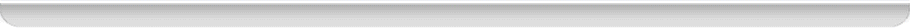# Team:UQ-Australia/Model## Modelling

Rationale

Modelling of our bioremediation system for the accumulation of mercury using E. coli is essential for a number of reasons.

Although it is difficult to create an accurate model of a biological system given the complexity of biological processes, an approximation of the system may be modelled. We have not yet had the opportunity to verify the accuracy of this model via 'wet lab' testing. Given the opportunity, we would improve upon the model and tailor it to the actual biological system.

Following the development and verification of a mathematical model, the model may be used to predict and inform future laboratory investigations and provide information as to the feasibility of practical applications for the research. In this case, the modelling could inform decisions made such as:

- the optical density (OD = A(600nm)) at which it is reasonable to test bioaccumulation

- the concentration of mercury which it is feasible to accumulate

- the need to over-express or inhibit the amount of regulatory proteins created in the cell

- the viability of using the bacterial system to purify mercury-contaminated water systems outside of the lab

Even though we did not get a chance to physically test our system, we understand the importance of modelling and the work below shall be used in conjunction with future experimental work on this system.

Diagrams

Initial Protein Concentrations

A matlab code (see Code section below) was created to determine the equilibrium concentrations of the relevant cellular proteins. The output of this code may be seen below:

The figure above shows the modelling used to calculate the equilibrium (stable) concentrations of cellular proteins. It may be seen that the complex - [MerR2.p2]- between the MerR dimer and plasmid 2 (p2) is at significantly lower concentration than [MerR]. This is due to the relatively low molar concentration of the low copy plasmid, p2 (a mere 5 copies per cell).

Mercury Uptake

Using the output (equilibrium) protein concentrations from the above diagram, a matlab code (see Code below was created to model the bioaccumulation of Mercury and transcription of the bacterial aggregation protein, Antigen43 (Ag43). The output of this code may be seen below:

This figure above shows the bioaccumulation of mercury to mM concentrations within the cell (see the total internal cytoplasmic concentration of mercury - [Hg]int - and the cytoplasmic concentration of unbound mercury - [Hg]free).

The potential of this technique is best seen by the decrease in the external mercury concentration - [Hg]ext.

Caveats

Many of the values for rate constants, etc. were unable to be determined from the literature. Hence, reasonable estimations have been made and are included in the matlab ".m" file in the Code section below.

Following testing in the lab, estimates for some of these values could be obtained, and the model could be updated.

Where to, now?

Before any more significant progress may be made on the model, experimental testing must be carried out. After the model is updated and validated, then it may be used to inform the design of future experimentation and (hopefully) in-field testing for the purification of mercury-contaminated systems.

Code

A system of simultaneous ordinary differential equations was developed and solved using matlab 'ODE45' solver.

The matlab code used for our modelling may be found here: media:UQ_Australia_2009_IGEM_modelling.m